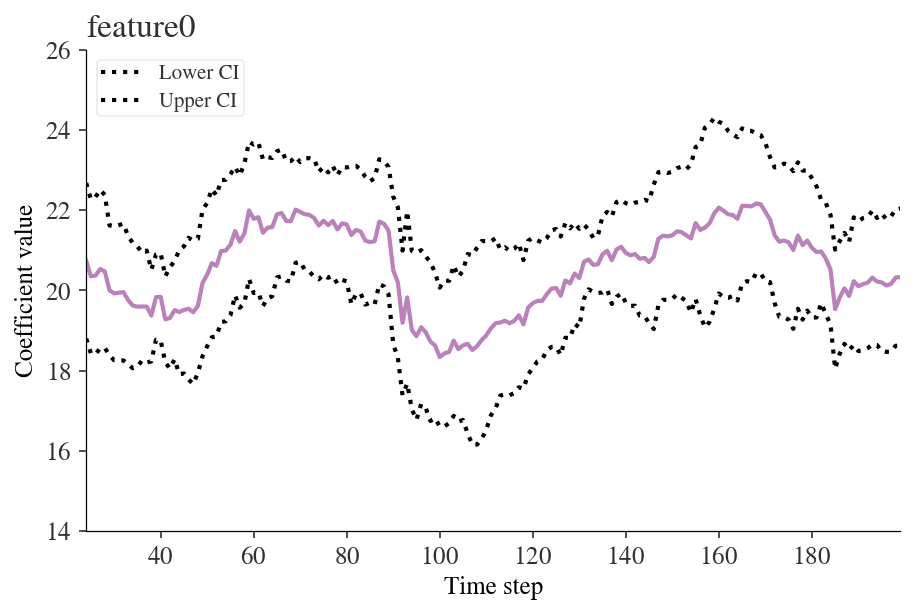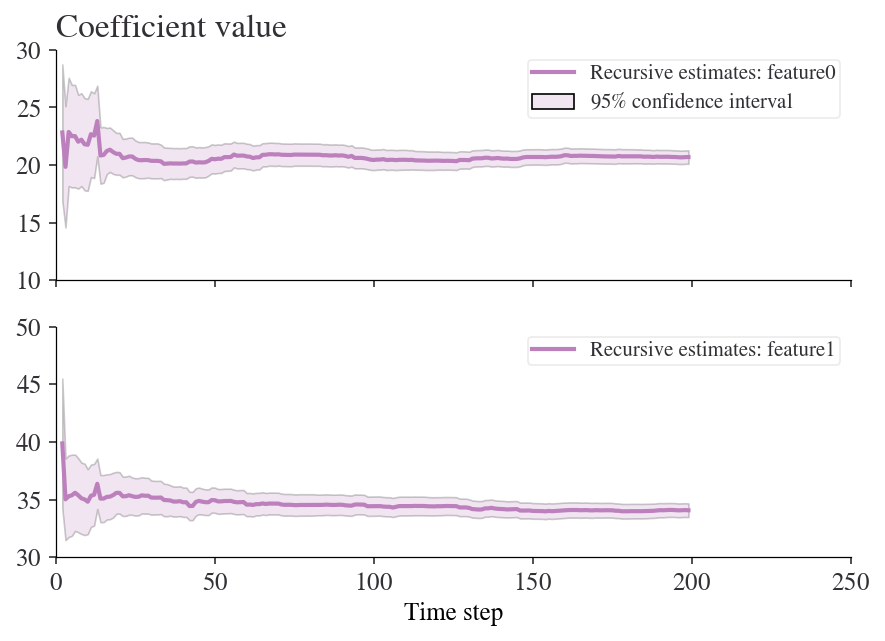# Rolling Regression

Rolling regressions are one of the simplest models for analysing changing relationships among variables overtime. They use linear regression but allow the data set used to change over time. In most linear regression models, parameters are assumed to be time-invariant and thus should not change overtime. Rolling regressions estimate model parameters using a fixed window of time over the entire data set. A larger sample size, or window, used will result in fewer parameter estimates but use more observations. For more information, see Base on Rolling.

## Keep in Mind

• When setting the width of your rolling regression you are also creating the starting position of your analysis given that it needs the a window sized amount of data begin.

## Also Consider

• An expanding window can be used where instead of a constantly changing fixed window, the regression starts with a predetermined time and then continually adds in other observations until the entire data set is used.

# Implementation

## Python

This example will make use of the statsmodels package, and some of the description of rolling regression has benefitted from the documentation of that package.

Rolling ordinary least squares applies OLS (ordinary least squares) across a fixed window of observations and then rolls (moves or slides) that window across the data set. The key parameter is window, which determines the number of observations used in each OLS regression.

First, let’s import the packages we’ll be using. If you don’t already have these installed, open up your terminal (aka command line) and use the command pip install packagename where ‘packagename’ is the package you want to install.

from statsmodels.regression.rolling import RollingOLS
import statsmodels.api as sm
from sklearn.datasets import make_regression
import matplotlib.pyplot as plt
import pandas as pd


Next we’ll create some random numbers to do our regression on

X, y = make_regression(n_samples=200, n_features=2, random_state=0, noise=4.0,
bias=0)
df = pd.DataFrame(X).rename(columns={0: 'feature0', 1: 'feature1'})
df['target'] = y

feature0 feature1 target
0 -0.955945 -0.345982 -36.740556
1 -1.225436 0.844363 7.190031
2 -0.692050 1.536377 44.389018
3 0.010500 1.785870 57.019515
4 -0.895467 0.386902 -16.088554

Now let’s fit the model using a formula and a window of 25 steps.

roll_reg = RollingOLS.from_formula('target ~ feature0 + feature1 -1', window=25, data=df)
model = roll_reg.fit()


Note that -1 just suppresses the intercept. We can see the parameters using model.params. Here are the params for time steps 20 to 30:

model.params[20:30]

feature0 feature1
20 NaN NaN
21 NaN NaN
22 NaN NaN
23 NaN NaN
24 20.736214 35.287604
25 20.351719 35.173493
26 20.368027 35.095621
27 20.532655 34.919468
28 20.470171 35.365235
29 20.002261 35.666997

Note that there aren’t parameters for entries between 0 and 23 because our window is 25 steps wide. We can easily look at how any of the coefficients are changing over time. Here’s an example for ‘feature0’.

fig = model.plot_recursive_coefficient(variables=['feature0'])
plt.xlabel('Time step')
plt.ylabel('Coefficient value')
plt.show()### Recursive ordinary least squares (aka expanding window rolling regression)

A rolling regression with an expanding (rather than moving) window is effectively a recursive least squares model. We can perform this kind of estimation using the RecursiveLS function from statsmodels. Let’s fit this to the whole dataset:

reg_rls = sm.RecursiveLS.from_formula(
'target ~ feature0 + feature1 -1', df)
model_rls = reg_rls.fit()
print(model_rls.summary())

                           Statespace Model Results
==============================================================================
Dep. Variable:                 target   No. Observations:                  200
Model:                    RecursiveLS   Log Likelihood                -570.923
Date:                Sun, 23 May 2021   R-squared:                       0.988
Time:                        17:05:03   AIC                           1145.847
Sample:                             0   BIC                           1152.444
- 200   HQIC                          1148.516
Covariance Type:            nonrobust   Scale                           17.413
==============================================================================
coef    std err          z      P>|z|      [0.025      0.975]
------------------------------------------------------------------------------
feature0      20.6872      0.296     69.927      0.000      20.107      21.267
feature1      34.0655      0.302    112.870      0.000      33.474      34.657
===================================================================================
Ljung-Box (Q):                       40.19   Jarque-Bera (JB):                 3.93
Prob(Q):                              0.46   Prob(JB):                         0.14
Heteroskedasticity (H):               1.17   Skew:                            -0.31
Prob(H) (two-sided):                  0.51   Kurtosis:                         3.31
===================================================================================

Warnings:
 Parameters and covariance matrix estimates are RLS estimates conditional on the entire sample.


But now we can look back at how the values of the coefficients changed in real time:

fig = model_rls.plot_recursive_coefficient(range(reg_rls.k_exog), legend_loc='upper right')
ax_list = fig.axes
for ax in ax_list:
ax.set_xlim(0, None)
ax_list[-1].set_xlabel('Time step')
ax_list.set_title('Coefficient value');## R

In R the rollRegres (one s, not two) package can compute rolling regressions while being able to specify the linear regression, window size, whether you want a rolling or expanding window, the minimum number of observations required in a window, and other options.

#Load in the package
library(rollRegres)


The data will be manually created where x can be interpreted as any independent variable over a fixed time period, and y is an outcome variable.

#Simulate data
set.seed(29132867)
n <- 200
p <- 2
X <- cbind(1, matrix(rnorm(p * n), ncol = p))
y <- drop(X %*% c(1, -1, 1)) + rnorm(n)
df_1 <- data.frame(y, X[, -1])

#Run the rolling regression (Rolling window)
roll_rolling <- roll_regres(y ~ X1, df_1, width = 25L, do_downdates = TRUE)

#Check the first 10 coefficients
roll_rolling$coefs %>% tail(25)  ## (Intercept) X1 ## 176 1.467609 -1.1887381 ## 177 1.561271 -1.0356878 ## 178 1.598559 -1.0142755 ## 179 1.615713 -0.8161185 ## 180 1.680253 -0.8814448 ## 181 1.593962 -0.8878947 ## 182 1.623287 -1.0246186 ## 183 1.596735 -1.0535530 ## 184 1.761971 -0.9466231 ## 185 1.703679 -0.8138562 ## 186 1.619849 -0.8948204 ## 187 1.729696 -0.9266218 ## 188 1.606376 -1.0624713 ## 189 1.676579 -0.9823355 ## 190 1.657288 -1.0377997 ## 191 1.579115 -1.1432474 ## 192 1.586334 -1.1829136 ## 193 1.393517 -1.1447650 ## 194 1.250160 -1.0244144 ## 195 1.243020 -1.0232251 ## 196 1.205068 -1.0201693 ## 197 1.273287 -0.9792404 ## 198 1.336927 -0.9346933 ## 199 1.343452 -0.9316855 ## 200 1.284072 -0.9379035  To demonstrate the point about a starting position for your analysis, the entries up to 24 are null because of the choice of window size. #Check the first 25 coefficients roll_rolling$coefs %>% head(25)

##    (Intercept)        X1
## 1           NA        NA
## 2           NA        NA
## 3           NA        NA
## 4           NA        NA
## 5           NA        NA
## 6           NA        NA
## 7           NA        NA
## 8           NA        NA
## 9           NA        NA
## 10          NA        NA
## 11          NA        NA
## 12          NA        NA
## 13          NA        NA
## 14          NA        NA
## 15          NA        NA
## 16          NA        NA
## 17          NA        NA
## 18          NA        NA
## 19          NA        NA
## 20          NA        NA
## 21          NA        NA
## 22          NA        NA
## 23          NA        NA
## 24          NA        NA
## 25    1.401621 -1.127908


Finally, here are the results when using an expanding window (also known as recursive regression)

#Run the rolling regression (Rolling window)
roll_expanding <- roll_regres(y ~ X1, df_1, width = 25L,do_downdates = FALSE)

#Check the last 10 coefficients
roll_expanding\$coefs %>% tail(10)

##     (Intercept)        X1
## 191    1.189651 -1.066285
## 192    1.196722 -1.077508
## 193    1.180968 -1.068470
## 194    1.178508 -1.064603
## 195    1.177739 -1.064419
## 196    1.170369 -1.063868
## 197    1.172466 -1.064678
## 198    1.179917 -1.056945
## 199    1.181876 -1.056017
## 200    1.178556 -1.054890